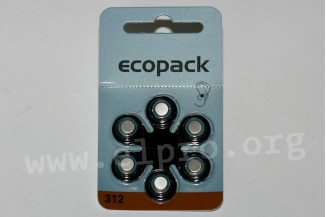## Categories### Varta button cell series Ecopack

• zinc-air button cells
• cross references PR 41: 312HPX = DA312 = 312DS = PR312H = HA312 = 312 AU = ZA312 = AC312 = A312
• cross references PR 48: 13HPX = DA13 = 13DS = PR13H = HA13 = 13 AU = ZA13 = AC13 = A13
• rated vol...

### Varta button cell series Ecopack

• zinc-air button cells
• cross references PR 41: 312HPX = DA312 = 312DS = PR312H = HA312 = 312 AU = ZA312 = AC312 = A312
• cross references PR 48: 13HPX = DA13 = 13DS = PR13H = HA13 = 13 AU = ZA13 = AC13 = A13
• rated voltage: 1,45V

6 button cells in one package.
Prices deal with one package, thus for 6 button cells.

> More